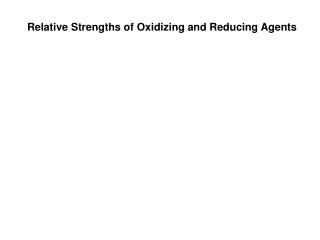# Relative Strengths of Oxidizing and Reducing Agents - PowerPoint PPT PresentationDownload PresentationRelative Strengths of Oxidizing and Reducing Agents

Relative Strengths of Oxidizing and Reducing Agents
Download Presentation## Relative Strengths of Oxidizing and Reducing Agents

- - - - - - - - - - - - - - - - - - - - - - - - - - - E N D - - - - - - - - - - - - - - - - - - - - - - - - - - -
##### Presentation Transcript

1. Relative Strengths of Oxidizing and Reducing Agents

2. metals: lose electrons and are good reducing agents. non-metals: gain electrons and are good oxidizing agents.

3. Brief Activity Series

4. Strong Reducing Agent.

5. Strong Oxidizing Agent

6. Li+(aq) + e-↔ Li(s) Eo = -3.04 V (non-spontaneous as written) Li+(aq) + e-→ Li(s) Eo = -3.04 V (non-spontaneous) Li+ is acting as a oxidizing agent (gaining an electron), but the negative sign shows this to be a non-spontaneous reaction. Li(s)→ Li+(aq) + e- Eo = +3.04 V (spontaneous) Li(s) is acting as a reducing agent (losing an electron) and the positive sign shows this to be a spontaneous reaction. So Li(s) makes a much better reducing agent than Li+(aq) makes as an oxidizing agent.

7. Li+(aq) + e-↔ Li(s) Eo = -3.04 V (non-spontaneous as written) Zn2+(aq) + 2e-↔ Zn(s) Eo = -0.76 V (non-spontaneous as written Li+(aq) + e-→ Li(s) Eo = -3.04 V (non-spontaneous) Li+ is acting as a oxidizing agent (gaining an electron), but the negative sign shows this to be a non-spontaneous reaction. Li(s)→ Li+(aq) + e- Eo = +3.04 V (spontaneous) Li(s) is acting as a reducing agent (losing an electron) and the positive sign shows this to be a spontaneous reaction. Zn2+(aq) + 2e-→ Zn(s) Eo = -0.76 V Zn2+ is acting as an oxidizing agent. Is it a stronger or weaker oxidizing agent than Li+? stronger,less negative Zn(s) → Zn2+(aq) + 2e- Eo = +0.76 V Zn(s) is acting as a reducing agent. Is it a stronger or weaker reducing agent than Li(s)? Weaker, +3.04 > +0.76

8. Li(s) can reduce Zn2+(aq) or Zn2+(aq) can oxidize Li(s) 2Li(s) + Zn2+(aq) → 2Li+(aq) + Zn(s) Eocell= +3.04 + -0.76 = +2.28 V What can be said about Mg(s) and Al3+(aq)? Mg(s) can reduce Al3+(aq) or Al3+(aq) can oxidize Mg(s). What about Al3+(aq) and Zn2+(aq)? Nothing will happen, they are both fully oxidized.

9. Cr(s) can give electrons (reduce) any of the ions below it.

10. Cd2+ can (oxidize) gain electrons from the elements above.